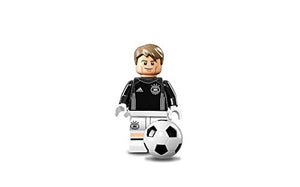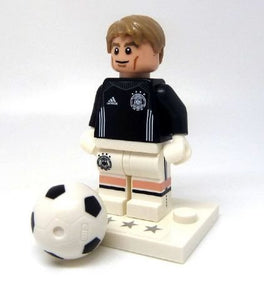# Lego 71014 - Minifigure Goalkeeper Manuel Neuer - No.1 From Dfb - The German Soccer Team (Open Bag)

Vendor
LEGO
Regular price
Sold out
Sale price
\$40.99
Quantity must be 1 or more

• L

• e

• g

• o

• 7

• 1

• 0

• 1

• 4

• -

• M

• i

• n

• i

• f

• i

• g

• u

• r

• e

• G

• o

• a

• l

• k

• e

• e

• p

• e

• r

• M

• a

• n

• u

• e

• l

• N

• e

• u

• e

• r

• -

• N

• o

• .

• 1

• f

• r

• o

• m

• D

• F

• B

• -

• T

• h

• e

• G

• e

• r

• m

• a

• n

• S

• o

• c

• c

• e

• r

• T

• e

• a

• m

• (

• o

• p

• e

• n

• b

• a

• g

• )

Lego 71014 - Minifigure Goalkeeper Manuel Neuer - No.1 from DFB - The German Soccer Team (open bag)